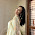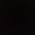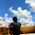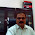## Sunday, 23 December 2012

### CBSE Class 7 - Maths - Comparing Quantities (NCERT Ex 8.3) - Profit and Loss and Simple Interest

Comparing Quantities
Profit & Loss and Simple Interest

Important points
Simple Interest
1. C.P. = Cost Price (Amount at which goods are purchased)
2. Sales Tax, VAT, cartage etc.are included in cost price.
3. S.P. = Selling Price (Amount at which goods are sold)
4. If SP > CP then it is Profit or Gain. Profit = SP - CP
5. If CP > SP then it is Loss. Loss = CP - SP
6. Profit of Loss per cent is always calculated on the cost price.

7. Similarly SP and CP can be computed as:

Simple Interest
8. Principal (P): Money borrowed or invested is called Principal or sum.
9. Interest: Additional money earned or paid on the invested money.
10. Rate (R%) =  Interest on Rs 100 for a year.
11. Simple Interest (SI) = Interest calculated uniformly on the original principal throughout the loan or invested period.
12. Amount = Principal + Interest
13. If P = principal, R = rate% per annum, T = number of years,
SI = P × R × T/100
NCERT EXERCISE 8.3

Q1: Tell what is the profit or loss in the following transactions. Also find profit per cent or loss per cent in each case.

(a) Gardening shears bought for Rs 250 and sold for Rs 325.
(b) A refrigerater bought for Rs 12,000 and sold at Rs 13,500.
(c) A cupboard bought for Rs 2,500 and sold at Rs 3,000.
(d) A skirt bought for Rs 250 and sold at Rs 150.

(a) SP = ₹325
CP = 250
Profit = 325 - 250 = 75
Profit% = (Profit/CP) × 100 = (75/250) × 100 = 30%

(b) SP = 13500
CP = 12000
SP > CP ⇒ Profit
Profit = 13500 - 12000 = 1500
Profit% = (Profit/CP) × 100 =(1500/12000) ×100 = 12.5%

(c) SP = 3000
CP = 2500
SP > CP ⇒ Profit
Profit = SP - CP = 3000 - 2500 = 500
Profit% = (Profit/CP) × 100 =  (500/2500) × 100 = 20%

(d) SP = 150
CP = 250
CP > SP  ⇒ Loss
Loss = CP - SP = 250 - 150 = 100
Loss% = (Loss/CP) × 100 =  (100/250) × 100 = 40%

Q2: Convert each part of the ratio to percentage:
(a) 3:1 (b) 2:3:5 (c) 1:4 (d) 1:2:5

(a) 3:1
Total parts = 3 + 1 = 4
1st part in % = (3/4) × 100 = 75%
2nd part in % = (1/4) × 100 = 25%

(b) 2:3:5
Total Parts = 2 + 3 + 5 = 10
1st part = (2/10) × 100 = 20%
2nd part = (3/10) × 100 = 30%
3rd part = (5/10) × 100 = 50%

(c) 1:4
Total Parts = 1 + 4 = 5
1st part = (1/5) × 100 = 20%
2nd part = (4/5) × 100 = 80%

(d) 1:2:5
Total parts = 1 + 2 + 5 = 8
1st part = (1/8) × 100 =12.5%
2nd part = (2/8) × 100 = 25%
3rd part = (5/8) × 100 =62.5%

Q3: The population of a city decreased from 25,000 to 24,500. Find the percentage decrease.

Answer: Initial population = 25000
Final population = 24500
Decrease in population = Final Population - Initial Population
= 24500 - 25000 = -500
(-ve value indicates decrease).

Percentage decrease = (decrease/initial value) × 100
= (-500/25000) × 100 = -2%

Q4: Arun bought a car for Rs 3,50,000. The next year, the price went upto Rs 3,70,000. What was the Percentage of price increase?

Answer: Initial price of car = 350000
Increased price of car = 370000
Actual Increase = Increased Value - Initial Value =   370000 - 350000
= 20000
Percent Increase = (Actual Increase/Initial Value)  × 100
= (20000/350000) × 100 = 40/7%

Q5: I buy a T.V. for Rs 10,000 and sell it at a profit of 20%. How much money do I get for it?

Answer: CP = 10000
Profit% = 20
SP = ?
SP = (100 + Profit%) × CP / 100 = (100+20) × 10000/100
= 120 × 100 = 12000

Q6: Juhi sells a washing machine for Rs 13,500. She loses 20% in the bargain. What was the price at which she bought it?

Answer: SP of washing machine = 13500
loss = 20%
CP = ?
CP =[100/(100 - loss%)] × SP = [100/(100 - 20)] × 13500
= (100/80) × 13500 = 16875

Q7: (i) Chalk contains calcium, carbon and oxygen in the ratio 10:3:12. Find the percentage of carbon in chalk.

(ii) If in a stick of chalk, carbon is 3g, what is the weight of the chalk stick?

(i)  Calcium:Carbon:Oxygen = 10:3:12
Total Parts = 10 + 3 + 12 = 25
Calcium % in Chalk = (10/25) × 100 = 40%
Carbon % in Chalk  =  (3/25) × 100 = 12%
Oxygen % in Chalk =   (12/25) × 100 = 48%

(ii) Weight of Carbon = 3g
Let the weight of chalk = n grams
Carbon % in Chalk  = 12%
⇒  12% of n =  3g
12/100 × n = 3
n = 3 × 100/12 = 25g

Q8: Amina buys a book for Rs 275 and sells it at a loss of 15%. How much does she sell it for?

Answer:  CP of the book = 275
Loss% = 15%
SP = [(100-loss%)/100] × CP = [(100 - 15)/100] × 275
= 85 × 275/100 = 23375/100 = 233.75

Q9: Find the amount to be paid at the end of 3 years in each case:
(a) Principal = Rs 1,200 at 12% p.a.
(b) Principal = Rs 7,500 at 5% p.a.

(a) Principal = 1200, R = 12%  T = 3years
SI = P×R×T/100 = 1200 × 12 × 3 /100 = 432
Amount = Principal + SI = 1200 + 432 = 1632

(b) Principal = 7500, R = 5% and T = 3 years
SI = P×R×T/100 =7500 × 5 × 3/ 100 = 1125
Amount = Principal + SI =7500 + 1125 = 8625

Q10: What rate gives Rs 280 as interest on a sum of Rs 56,000 in 2 years?

Answer:  Principal = 56000
SI = 280
Time (T) = 2 years
Rate (R) = ?
R = (SI × 100)/ (P × T)
= (280 × 100) / (56000 × 2) = 28/(56 x 2) = 1/4 = 0.25%

Q11: If Meena gives an interest of Rs 45 for one year at 9% rate p.a.. What is the sum she has borrowed?

Answer: SI = 45
Rate = 9% p.a.
Time = 1 year
Principal = ?
P = (SI × 100)/ (R × T) = (45 × 100)/ (9 × 1)  = 500

Misc. Questions

Q12: By selling California apples at Rs 384 a kilogram, a shopkeeper incurs a loss of 4%. At what price mush he sell so that he get a profit of 10%

Answer: SP  = 384  and loss = 4%
CP = [100/(100 - loss%)] × SP
∴  CP = [100/(100 - 4)] × 384
CP = 100/96  × 384  = 100 × 4 = 400

CP = 400
Gain = 10%
SP = [(100+gain%)/100] × CP = [(100+10)/100] × 400
= 110/100 × 400 = 440

(In progress...)1.this is helpful but give harder sums

2.Its good like to see more

3.it is a little hard if they would have given the sums it would be better i liked it
:-p

4.easy to understand. specially for a school student

5.This comment has been removed by the author.

6.This comment has been removed by the author.

7.nice and correct answers

8.very helpful and understanding
but would be better if given harder sums

9.Good but only is one hard sum last one